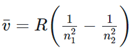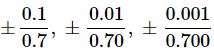# Assertion & Reason Type Questions: Physical World Notes | Study Physics Class 11 - NEET

## NEET: Assertion & Reason Type Questions: Physical World Notes | Study Physics Class 11 - NEET

The document Assertion & Reason Type Questions: Physical World Notes | Study Physics Class 11 - NEET is a part of the NEET Course Physics Class 11.
All you need of NEET at this link: NEET

For following question, two statements are given-one labelled Assertion- (A) and the other labeled Reason (R). Select the correct answer to these questions from the codes (a), (b), (c) and (d) as given below.
Question 1:
Assertion (A): Energy is a scalar quantity.
Reason (R): All conserved quantities are scalars.
(a) Both A and R are true and R is the correct explanation of A
(b) Both A and R are true but R is NOT the correct explanation of A
(c) A is true but R is false
(d) A is false and R is also false

Assertion is true since energy is a scalar quantity. But all conserved quantities are not necessarily scalars. The total linear momentum and the total angular momentum (both vectors) of an isolated system are also conserved quantities. So, the reason is not true.

Question 2:
Assertion (A): Classical Physics mainly includes subjects like Mechanics, Electrodynamics, Optics and Thermodynamics.
Reason (R): The macroscopic domain includes phenomena at the laboratory, terrestrial and astronomical scales.
(a) Both A and R are true and R is the correct explanation of A
(b) Both A and R are true but R is NOT the correct explanation of A
(c) A is true but R is false
(d) A is false and R is also false

Assertion is true since classical physics mainly includes subjects like Mechanics, Electrodynamics, Optics and Thermodynamics. Mechanics is concerned with the motion (or equilibrium) of particles, rigid and deformable bodies, and general systems of particles. Electrodynamics deals with electric and magnetic phenomena associated with charged and magnetic bodies. Optics deals with the phenomena involving light. The working of telescopes and microscopes, colours exhibited by thin films, etc., are topics in optics. modyn Tahmerics deals with systems in macroscopic equilibrium and is concerned with changes in internal energy, temperature, entropy, etc., of the system through external work and transfer of heat. So all these are in macroscopic domain. So reason is also true and properly explains the assertion.

Question 3:
Assertion (A): Wireless communication technology that followed the discovery of the basic laws of electricity and magnetism.
Reason (R): Technology gives rise to new physics.
(a) Both A and R are true and R is the correct explanation of A
(b) Both A and R are true but R is NOT the correct explanation of A
(c) A is true but R is false
(d) A is false and R is also false

Assertion is true since the basic laws of electricity and magnetism were discovered first and then the wireless communication technology developed. Reason is also true that sometimes technology gives rise to new physics. But it is not applicable to the assertion.

Question 4:
Assertion: Units of Rydberg constant R are m-1
Reason: It follows from Bohr’s formula, where the symbols have their usual meaning.
(a) If both the assertion and the reason are true and the reason is a correct explanation of the assertion
(b) If both the assertion and reason are true but the reason is not a correct explanation of the assertion
(c) If the assertion is true but the reason is false
(d) If both the assertion and reason are false

We Know that Rydberg Equation
1/λ = RH (1/H21 - 1/H22)
[RH] = [L-1]
Unit of RH is m-1

Question 5:
Assertion: Parallex method cannot be used for measuring distances of stars more than 100 light years away.
Reason: Because parallex angle reduces so much that it cannot be measured accurately.
(a) If both the assertion and the reason are true and the reason is a correct explanation of the assertion
(b) If both the assertion and reason are true but the reason is not a correct explanation of the assertion
(c) If the assertion is true but the reason is false
(d) If both the assertion and reason are false

Parallax angle θ = I/r
As distance of (r ) increases, θ reduces.
Measurement becomes inaccurate.

Question 6:
Assertion: Number of significant figures in 0.005 is one and that in 0.500 is three.
Reason: This is because zeros are not significant.
(a) If both the assertion and the reason are true and the reason is a correct explanation of the assertion
(b) If both the assertion and reason are true but the reason is not a correct explanation of the assertion
(c) If the assertion is true but the reason is false
(d) If both the assertion and reason are false

In a number less then one, zero between the decimal point and first non zero digit are not significant. But zeros to the right of last non zero digit are significant. Statement-1 is true, but statement -2 is false.

Question 7:
Assertion: Out of three measurements l = 0.7 m; l = 0.70 m and l = 0.700 m, the last one is most accurate.
Reason: In every measurement, only the last significant digit is not accurately known.

(a) If both the assertion and the reason are true and the reason is a correct explanation of the assertion
(b) If both the assertion and reason are true but the reason is not a correct explanation of the assertion
(c) If the assertion is true but the reason is false
(d) If both the assertion and reason are false

Fractional error in the three measurements respectively areQuestion 8:
Assertion: Mass, length and time are fundamental physical quantities.
Reason: They are independent of each other.

(a) If both the assertion and the reason are true and the reason is a correct explanation of the assertion
(b) If both the assertion and reason are true but the reason is not a correct explanation of the assertion
(c) If the assertion is true but the reason is false
(d) If both the assertion and reason are false

Mass, length and time are fundamental physical quantities as they are not derived from each other or we can say they are independent of each other.

Question 9:
Assertion: Density is a derived physical quantity.
Reason: Density cannot be derived from the fundamental physical quantities.
(a) If both the assertion and the reason are true and the reason is a correct explanation of the assertion
(b) If both the assertion and reason are true but the reason is not a correct explanation of the assertion
(c) If the assertion is true but the reason is false
(d) If both the assertion and reason are false

Because density can be derived from fundamental quantities.

Question 10:
Assertion: Nowadays a standard metre is defined in terms of the wavelength of light.
Reason: Light has no relation with length.

(a) If both the assertion and the reason are true and the reason is a correct explanation of the assertion
(b) If both the assertion and reason are true but the reason is not a correct explanation of the assertion
(c) If the assertion is true but the reason is false
(d) If both the assertion and reason are false

Assertion statement is true that a standard metre is defined as in terms of wavelength of light. Velocity of light v = νλ
where λ is the wavelength of light and so light has relation with length parameter.
Thus reason statement is wrong.

The document Assertion & Reason Type Questions: Physical World Notes | Study Physics Class 11 - NEET is a part of the NEET Course Physics Class 11.
All you need of NEET at this link: NEETUse Code STAYHOME200 and get INR 200 additional OFF

## Physics Class 11

122 videos|449 docs|210 tests

### How to Prepare for NEET

Read our guide to prepare for NEET which is created by Toppers & the best Teachers

Track your progress, build streaks, highlight & save important lessons and more!

,

,

,

,

,

,

,

,

,

,

,

,

,

,

,

,

,

,

,

,

,

;